# Atomic Mass Atomic mass or atomic weight is

• Slides: 33Atomic Mass • Atomic mass or atomic weight is the average mass of atoms of an element, calculated using the relative abundance of isotopes in a naturally-occuring element.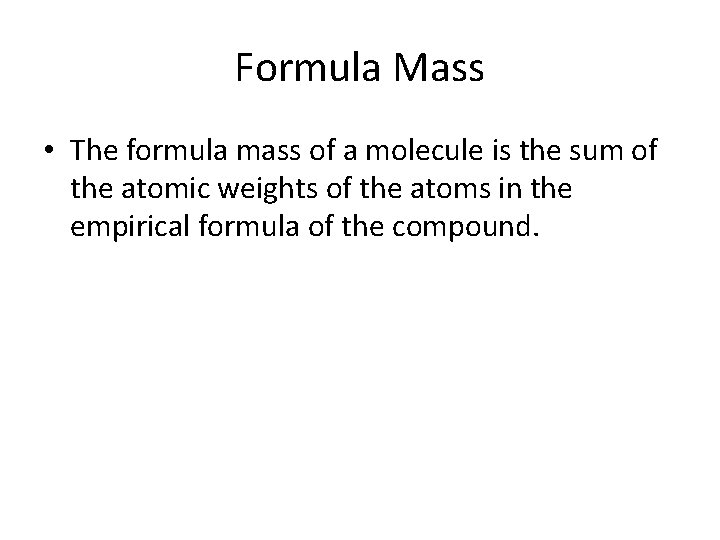Formula Mass • The formula mass of a molecule is the sum of the atomic weights of the atoms in the empirical formula of the compound.Half Life • is the time required for a quantity to fall to half its value as measured at the beginning of the time periodBeta Particle • are high-energy, high-speed electrons emitted by certain types of radioactive nucleiGamma Particle • Electromagnetic radiation emitted by radioactive decay and having energies in a range from ten thousand (104) to ten million (107) electron volts.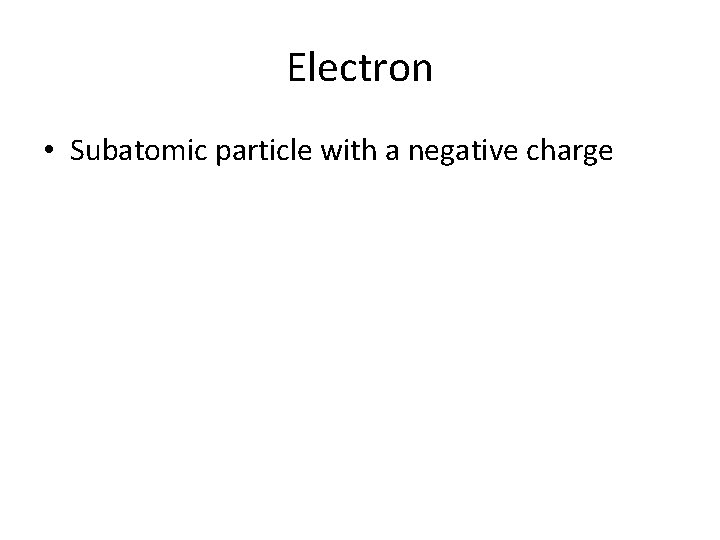Electron • Subatomic particle with a negative chargeIsotope • Atoms with the same number of protons, but differing number of neutrons. Different forms of a single element.Energy Level • The energy level denoted by the principal quantum number. The first elemebt in a period of the Periodic table introduces a new principal energy level.Periodic Table • Tabular arrangement of the chemical elements, organized on the basis of their atomic numbers, electron configurations, and recurring chemical properties.Neutron • Subatomic particle with a neutral charge found in the nucleus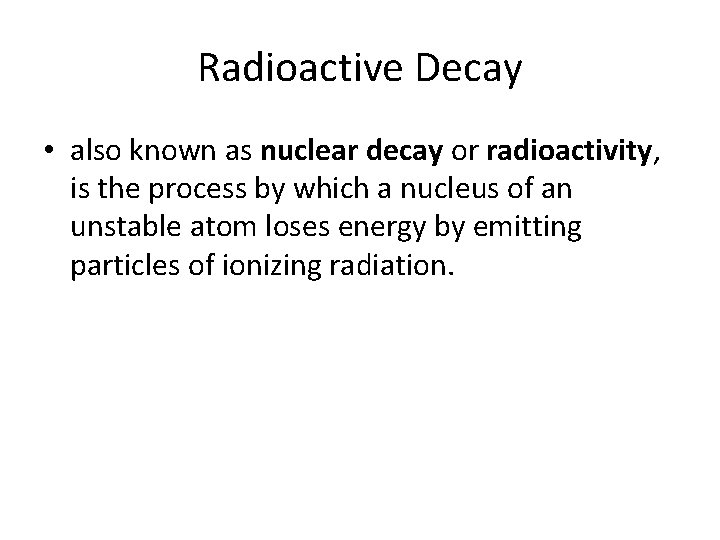Radioactive Decay • also known as nuclear decay or radioactivity, is the process by which a nucleus of an unstable atom loses energy by emitting particles of ionizing radiation.Atomic Structure • The atom is a basic unit of matter that consists of a dense, central nucleus surrounded by a cloud of negatively charged electrons.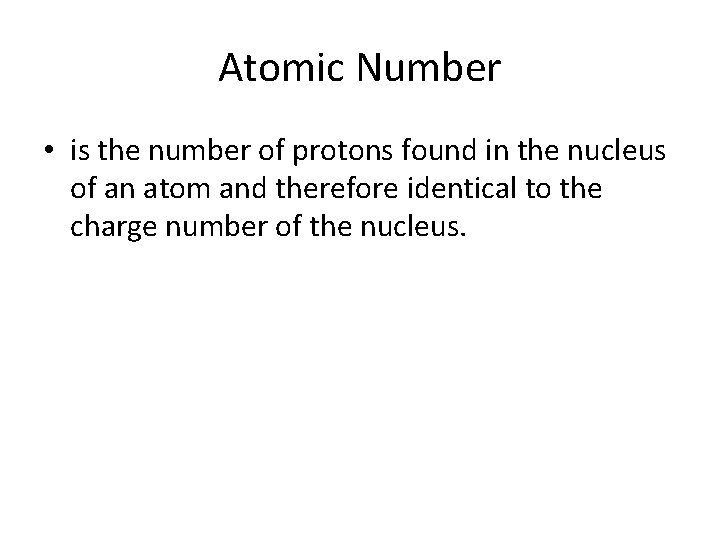Atomic Number • is the number of protons found in the nucleus of an atom and therefore identical to the charge number of the nucleus.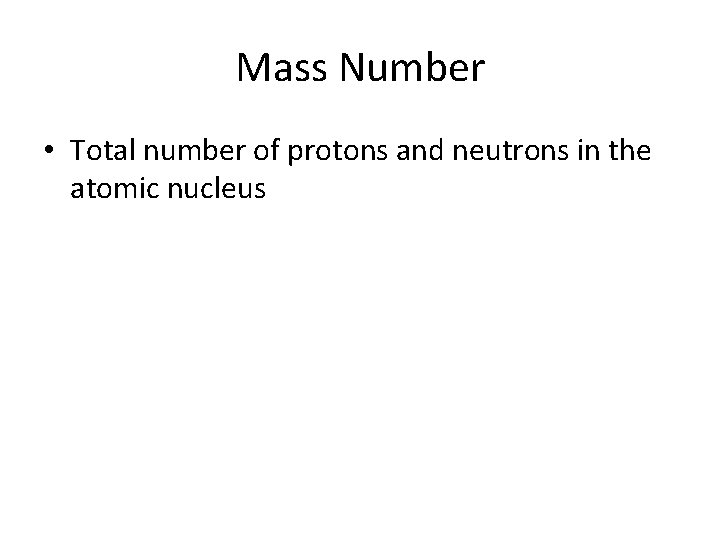Mass Number • Total number of protons and neutrons in the atomic nucleus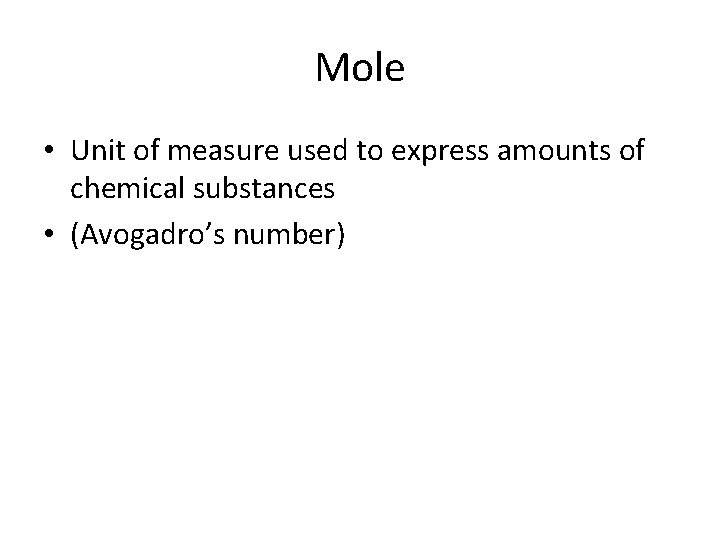Mole • Unit of measure used to express amounts of chemical substances • (Avogadro’s number)Atom • basic unit of matter that consists of a dense central nucleus surrounded by a cloud of negatively charged electrons.Percent Composition • The percent composition of a component in a compound is the percent of the total mass of the compound that is due to that component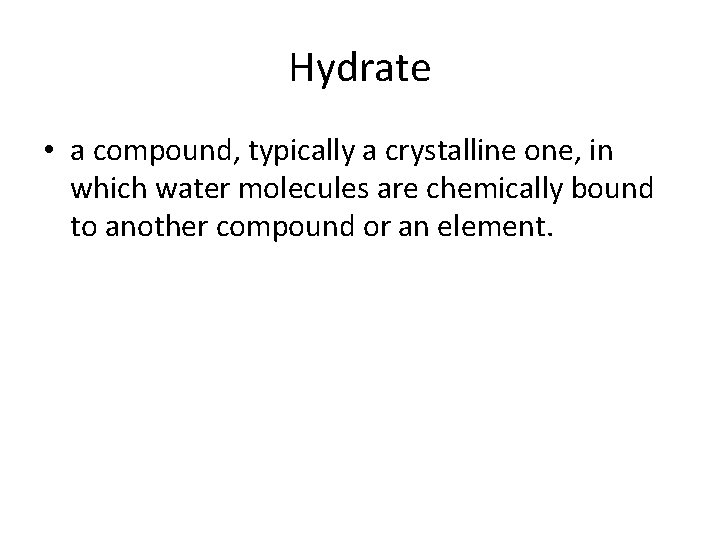Hydrate • a compound, typically a crystalline one, in which water molecules are chemically bound to another compound or an element.Anion • Negatively charged ionCation • Positively charged ion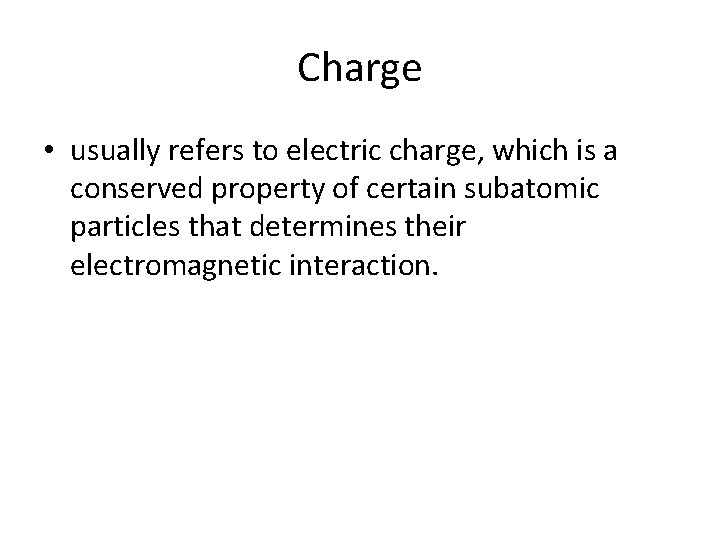Charge • usually refers to electric charge, which is a conserved property of certain subatomic particles that determines their electromagnetic interaction.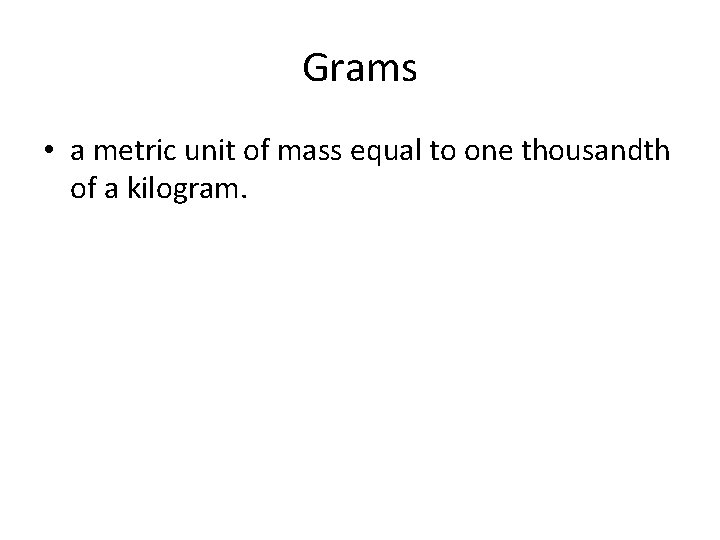Grams • a metric unit of mass equal to one thousandth of a kilogram.Electron Cloud • The region of negative charge surrounding an atomic nucleus that is associated with an atomic orbital.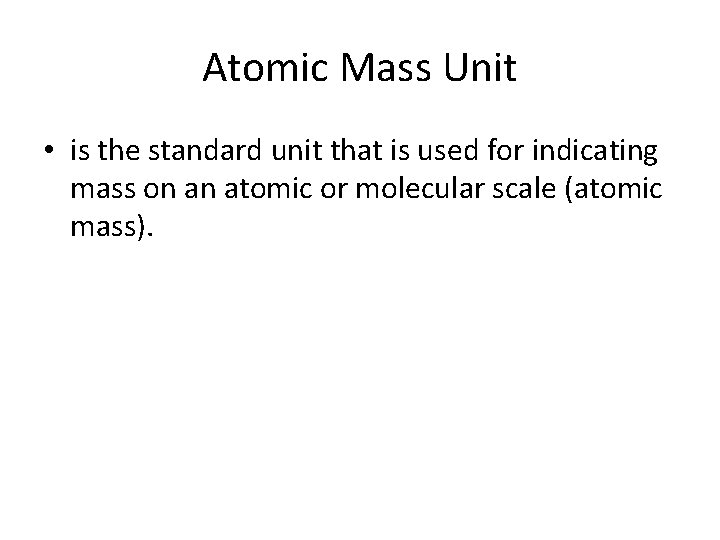Atomic Mass Unit • is the standard unit that is used for indicating mass on an atomic or molecular scale (atomic mass).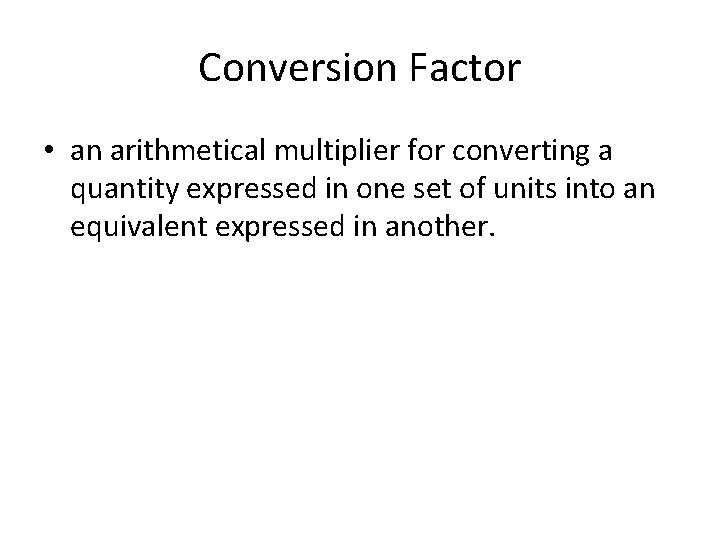Conversion Factor • an arithmetical multiplier for converting a quantity expressed in one set of units into an equivalent expressed in another.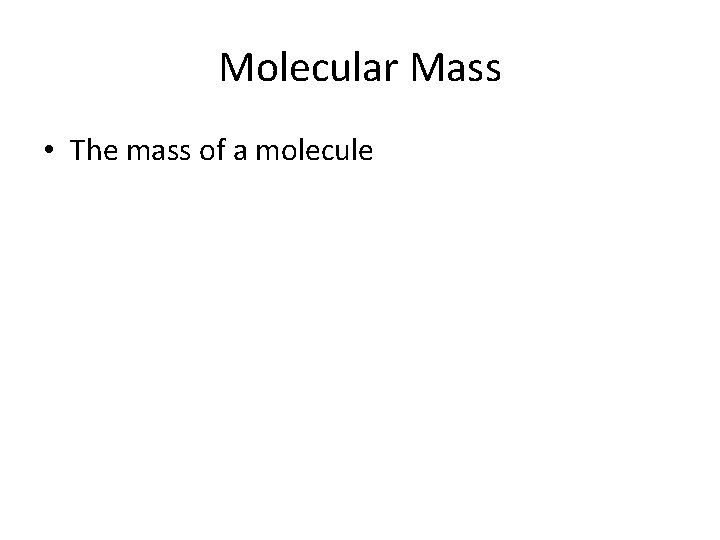Molecular Mass • The mass of a molecule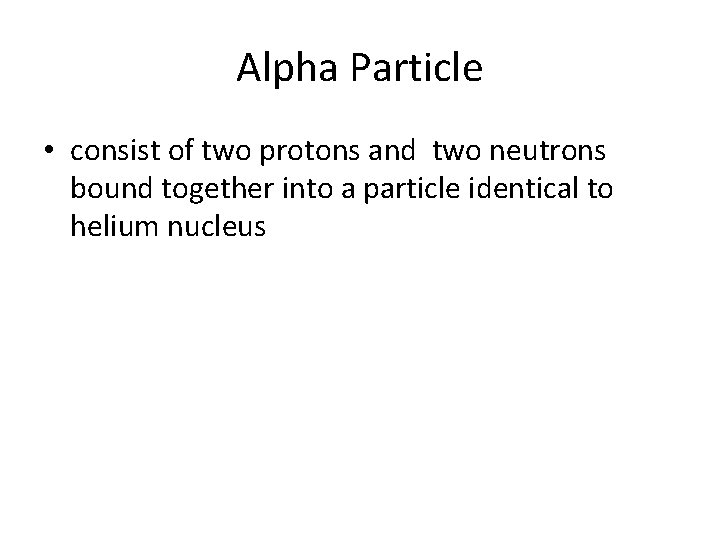Alpha Particle • consist of two protons and two neutrons bound together into a particle identical to helium nucleus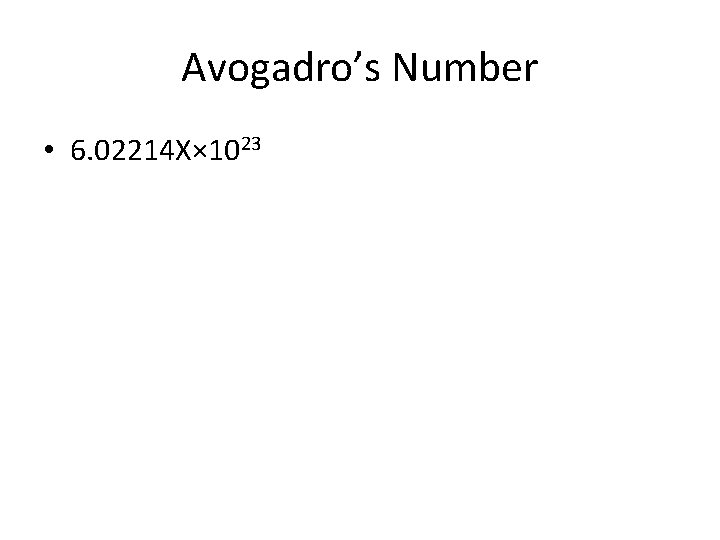Avogadro’s Number • 6. 02214 X× 1023Dalton’s Atomic Theory • 1) All matter is made of atoms. Atoms are indivisible and indestructible. • 2) All atoms of a given element are identical in mass and properties • 3) Compounds are formed by a combination of two or more different kinds of atoms. • 4) A chemical reaction is a rearrangement of atoms.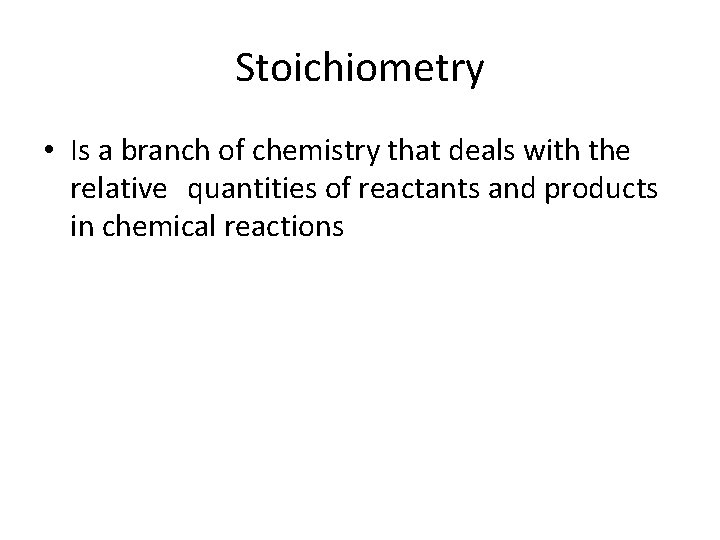Stoichiometry • Is a branch of chemistry that deals with the relative quantities of reactants and products in chemical reactionsIon • an atom or molecule with a net electric charge due to the loss or gain of one or more electronProton • A subatomic particle occurring in the nuclei with a positive chargeEmpirical Formula • a formula giving the proportions of the elements present in a compound but not the actual numbers or arrangement of atoms.